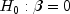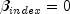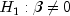JMSLTM Numerical Library 7.2.0
com.imsl.stat

## Class StepwiseRegression.CoefficientTTests

• All Implemented Interfaces:
Serializable
Enclosing class:
StepwiseRegression

```public class StepwiseRegression.CoefficientTTests
extends Object
implements Serializable```
`CoefficientTTests` contains statistics related to the student-t test, for each regression coefficient.
Serialized Form
• ### Method Summary

Methods
Modifier and Type Method and Description
`double` `getCoefficient(int index)`
Returns the estimate for a coefficient of the independent variable.
`double` `getPValue(int index)`
Returns the p-value for the two-sided testvs.
`double` `getStandardError(int index)`
Returns the estimated standard error for a coefficient estimate.
`double` `getTStatistic(int index)`
Returns the student-t test statistic for testing the i-th coefficient equal to zero ().
• ### Methods inherited from class java.lang.Object

`clone, equals, finalize, getClass, hashCode, notify, notifyAll, toString, wait, wait, wait`
• ### Method Detail

• #### getCoefficient

`public double getCoefficient(int index)`
Returns the estimate for a coefficient of the independent variable.
Parameters:
`index` - an `int` which specifies the index of the coefficient whose estimate is to be returned. ``` index``` must be between 1 and the number of independent variables.
Returns:
a `double` which contains the estimate for the coefficient.
• #### getPValue

`public double getPValue(int index)`
Returns the p-value for the two-sided testvs..
Parameters:
`index` - an `int` which specifies the index of the coefficient whose p-value is to be returned. `index` must be between 1 and the number of independent variables.
Returns:
a `double` which contains the estimated p-value for the coefficient.
• #### getStandardError

`public double getStandardError(int index)`
Returns the estimated standard error for a coefficient estimate.
Parameters:
`index` - an `int` which specifies the index of the coefficient whose standard error estimate is to be returned. `index` must be between 1 and the number of independent variables.
Returns:
a `double` which contains the estimated standard error for the coefficient.
• #### getTStatistic

`public double getTStatistic(int index)`
Returns the student-t test statistic for testing the i-th coefficient equal to zero ().
Parameters:
`index` - an `int` which specifies the index of the coefficient whose t-test statistic is to be returned. `index` must be between 1 and the number of independent variables.
Returns:
a `double` which contains the estimated t-test statistic for the coefficient.
JMSLTM Numerical Library 7.2.0